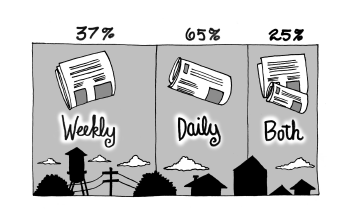### Home > INT2 > Chapter 7 > Lesson 7.2.3 > Problem7-107

7-107.In a certain small town, $65\%$ of the households subscribe to the daily paper, $37\%$ subscribe to the weekly local paper, and $25\%$ subscribe to both papers.

1. Make a two-way table to represent this data.

 Daily Not Daily Weekly local $25\%$ $37\%$ Not weekly local $65\%$ $100\%$
2. If a household is selected at random, what is the probability that it subscribes to at least one of the two papers? Shade these areas in your table.

 Daily Not Daily Weekly local $25\%$ $\boldsymbol{12\%}$ $37\%$ Not weekly local $\boldsymbol{40\%}$ $\boldsymbol{23\%}$ $\boldsymbol{63\%}$ $65\%$ $\boldsymbol{35\%}$ $100\%$

3. Charlie’s neighbor subscribes to at least one paper. What is the probability that he receives the daily paper?

$\frac{25 + 40}{77} \approx 84.4\%$